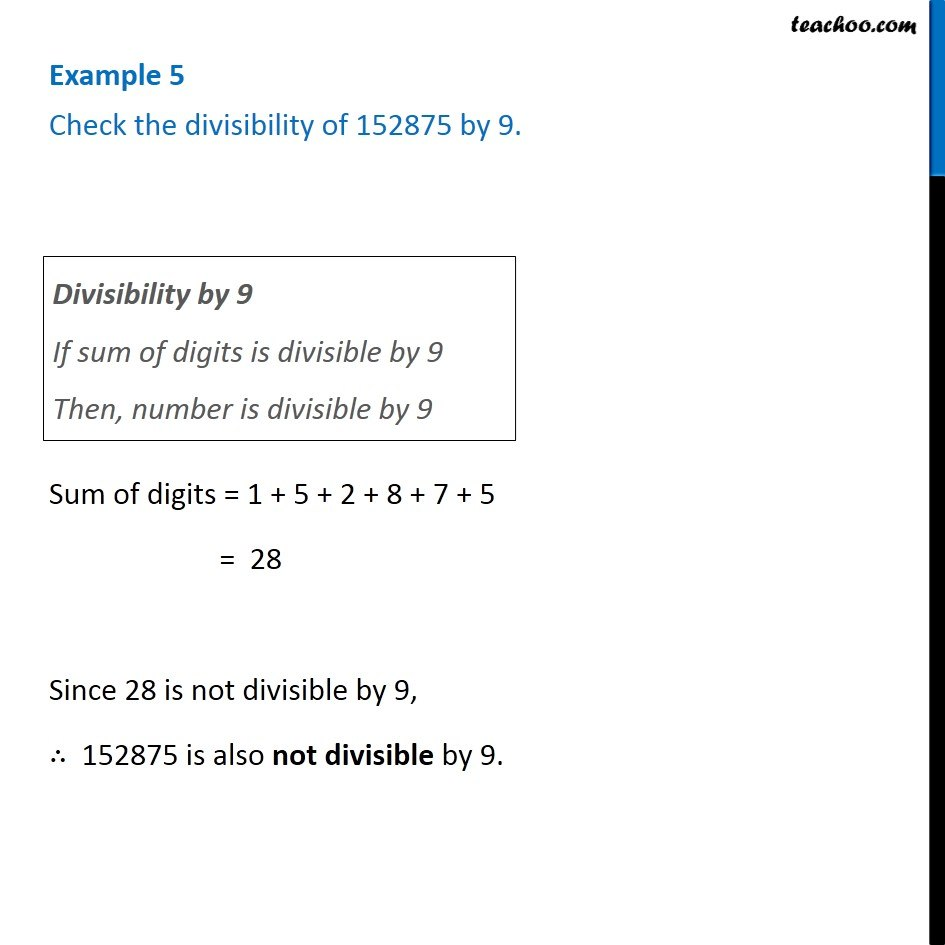Examples

Chapter 16 Class 8 Playing with Numbers
Serial order wiseGet live Maths 1-on-1 Classs - Class 6 to 12

### Transcript

Example 5 Check the divisibility of 152875 by 9. Divisibility by 9 If sum of digits is divisible by 9 Then, number is divisible by 9 Sum of digits = 1 + 5 + 2 + 8 + 7 + 5 = 28 Since 28 is not divisible by 9, ∴ 152875 is also not divisible by 9.